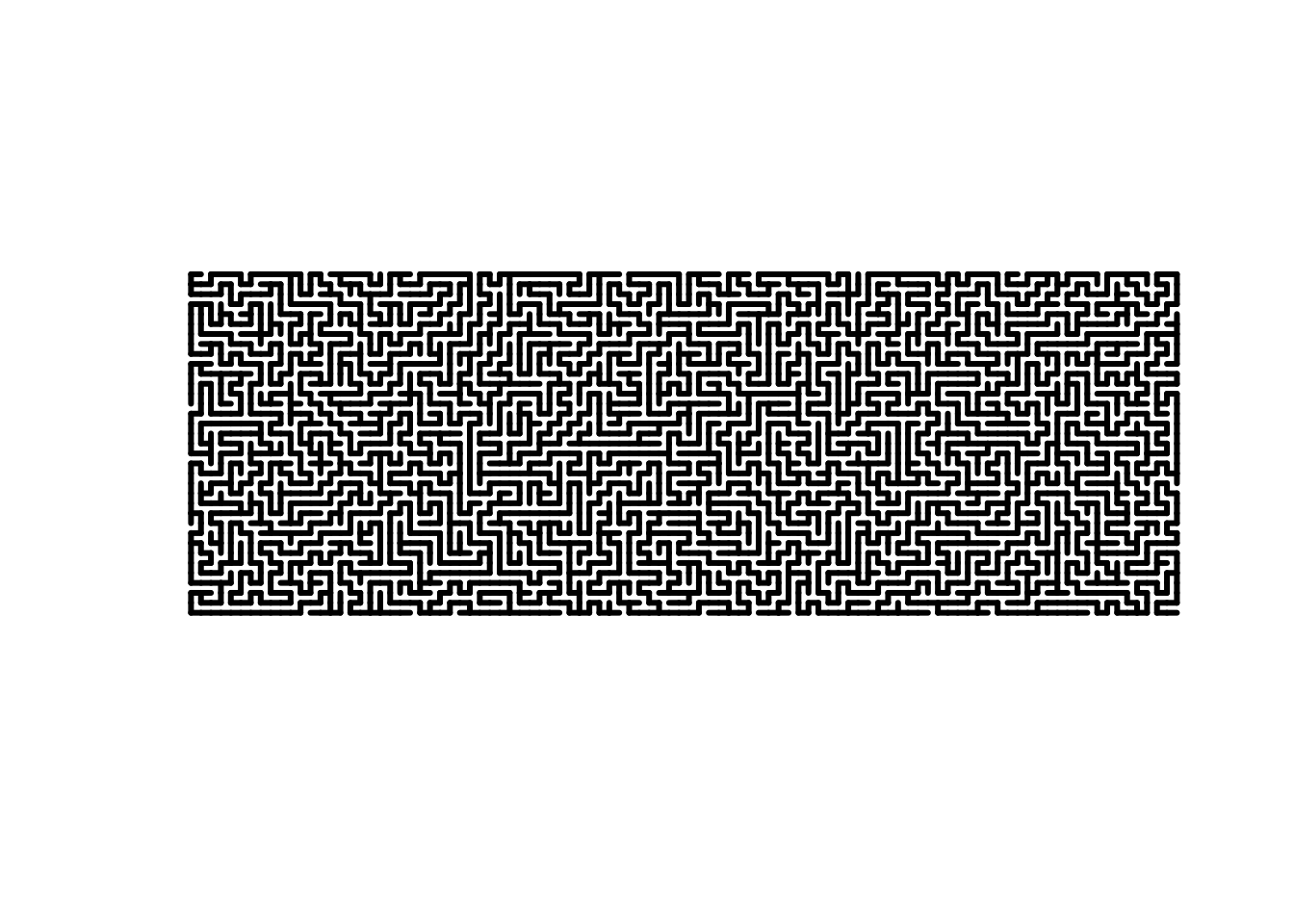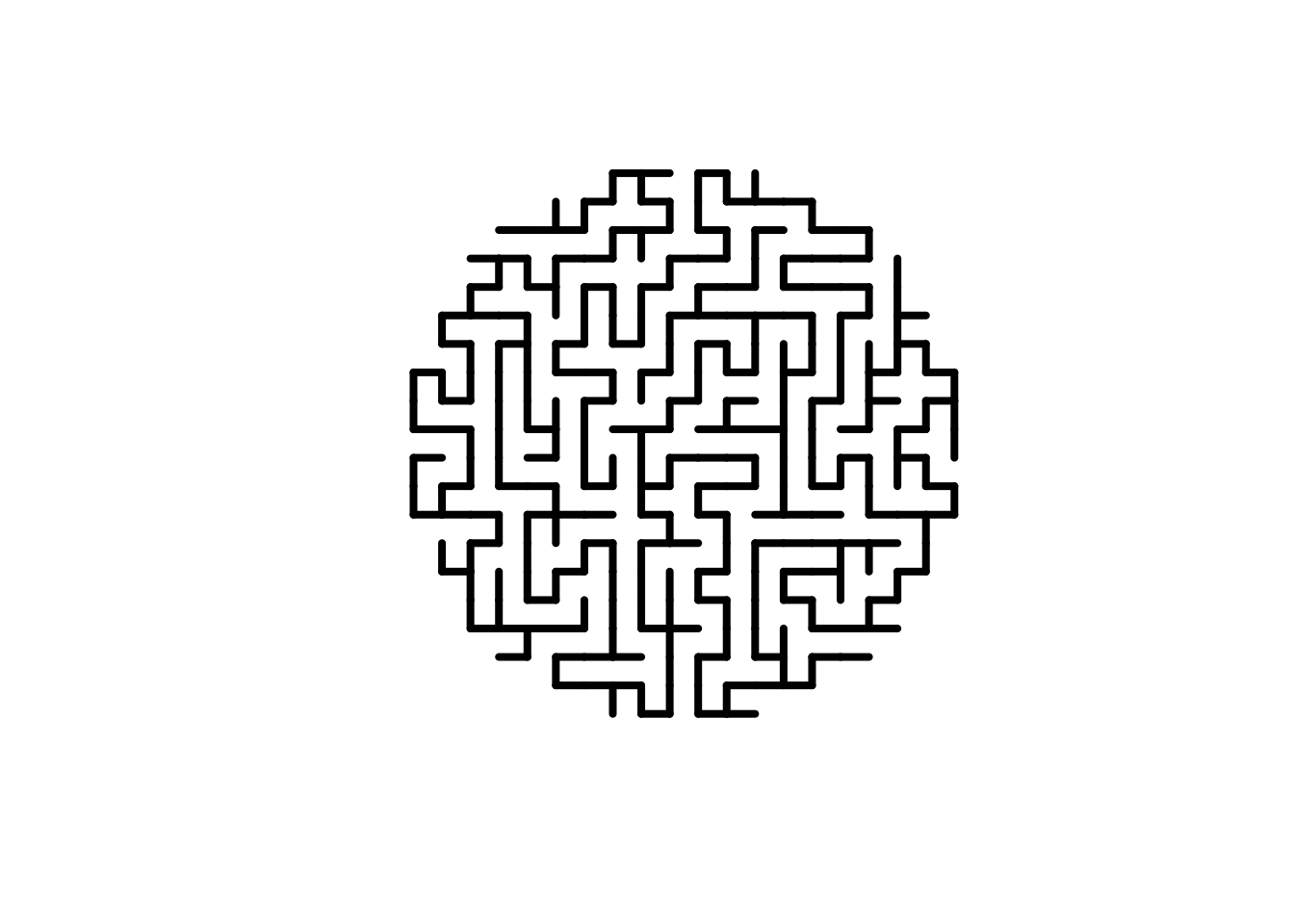Hi! Thanks for checking out the `mazing` package! In this document, I’ll provide examples of some of the things you can do with the package and hopefully inspire you to make some cool mazes of your own.

## Creating mazes

The most basic functionality of `mazing` is producing a rectangular maze, which we do with the `maze` function.

``````m <- maze(35,100)
plot(m, lwd = 3)``````We can also produce mazes from existing binary matrices. This allows us to create mazes in a wider variety of shapes!

``````mat <- matrix(1, 20, 20)
for(i in 1:nrow(mat)){
for(j in 1:ncol(mat)){
if((i-10.5)^2+(j-10.5)^2 > 100){
mat[i,j] <- 0
}
}
}
m <- as.maze(mat)
plot(m, lwd = 4)``````This is also how I produced the hexagonal maze for the sticker: